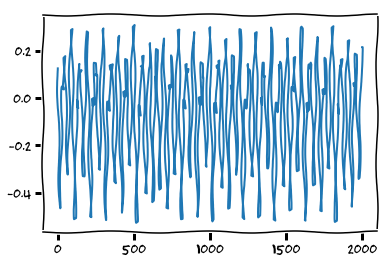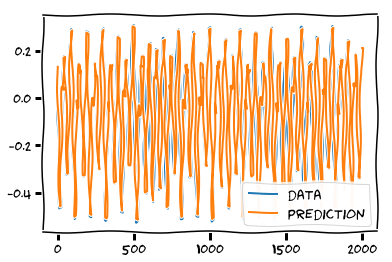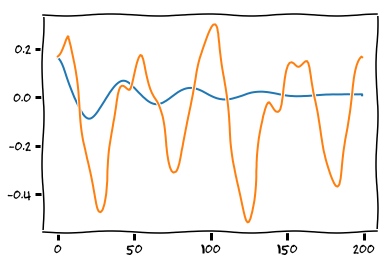# Recurrent Neural Networks in Theano¶

Credits: Forked from summerschool2015 by mila-udem

First, we import some dependencies:

In :
%matplotlib inline
from synthetic import mackey_glass
import matplotlib.pyplot as plt
import theano
import theano.tensor as T
import numpy
plt.xkcd()

floatX = theano.config.floatX


We now define a class that uses scan to initialize an RNN and apply it to a sequence of data vectors. The constructor initializes the shared variables after which the instance can be called on a symbolic variable to construct an RNN graph. Note that this class only handles the computation of the hidden layer activations. We'll define a set of output weights later.

In :
class SimpleRNN(object):
def __init__(self, input_dim, recurrent_dim):
w_xh = numpy.random.normal(0, .01, (input_dim, recurrent_dim))
w_hh = numpy.random.normal(0, .02, (recurrent_dim, recurrent_dim))
self.w_xh = theano.shared(numpy.asarray(w_xh, dtype=floatX), name='w_xh')
self.w_hh = theano.shared(numpy.asarray(w_hh, dtype=floatX), name='w_hh')
self.b_h = theano.shared(numpy.zeros((recurrent_dim,), dtype=floatX), name='b_h')
self.parameters = [self.w_xh, self.w_hh, self.b_h]

def _step(self, input_t, previous):
return T.tanh(T.dot(previous, self.w_hh) + input_t)

def __call__(self, x):
x_w_xh = T.dot(x, self.w_xh) + self.b_h
sequences=[x_w_xh],
outputs_info=[T.zeros_like(self.b_h)])
return result


For visualization purposes and to keep the optimization time managable, we will train the RNN on a short synthetic chaotic time series. Let's first have a look at the data:

In :
data = numpy.asarray(mackey_glass(2000), dtype=floatX)
plt.plot(data)
plt.show()
data_train = data[:1500]
data_val = data[1500:]To train an RNN model on this sequences, we need to generate a theano graph that computes the cost and its gradient. In this case, the task will be to predict the next time step and the error objective will be the mean squared error (MSE). We also need to define shared variables for the output weights. Finally, we also add a regularization term to the cost.

In :
w_ho_np = numpy.random.normal(0, .01, (15, 1))
w_ho = theano.shared(numpy.asarray(w_ho_np, dtype=floatX), name='w_ho')
b_o = theano.shared(numpy.zeros((1,), dtype=floatX), name='b_o')

x = T.matrix('x')
my_rnn = SimpleRNN(1, 15)
hidden = my_rnn(x)
prediction = T.dot(hidden, w_ho) + b_o
parameters = my_rnn.parameters + [w_ho, b_o]
l2 = sum((p**2).sum() for p in parameters)
mse = T.mean((prediction[:-1] - x[1:])**2)
cost = mse + .0001 * l2


We now compile the function that will update the parameters of the model using gradient descent.

In :
lr = .3
updates = [(par, par - lr * gra) for par, gra in zip(parameters, gradient)]
get_cost = theano.function([x], mse)
predict = theano.function([x], prediction)
get_hidden = theano.function([x], hidden)


We can now train the network by supplying this function with our data and calling it repeatedly.

In :
for i in range(1001):
mse_train = update_model(data_train)

if i % 100 == 0:
mse_val = get_cost(data_val)
print('Epoch {}: train mse: {}    validation mse: {}'.format(i, mse_train, mse_val))

Epoch 0: train mse: 0.05159830456706826    validation mse: 0.04688619396459131
Epoch 100: train mse: 0.03308586204754072    validation mse: 0.031912791487249084
Epoch 200: train mse: 0.002231280627935095    validation mse: 0.002057841132534526
Epoch 300: train mse: 0.0018355755403003256    validation mse: 0.0016508816899916982
Epoch 400: train mse: 0.0016013576287368058    validation mse: 0.0014066188731226932
Epoch 500: train mse: 0.0014443176507955217    validation mse: 0.0012405799393282923
Epoch 600: train mse: 0.001330610671787295    validation mse: 0.0011186985124206118
Epoch 700: train mse: 0.001243807104666937    validation mse: 0.0010243720769920668
Epoch 800: train mse: 0.0011748882250026316    validation mse: 0.0009484523467887362
Epoch 900: train mse: 0.0011184538218755669    validation mse: 0.0008854402691881776
Epoch 1000: train mse: 0.001071052484828377    validation mse: 0.0008318068096166963


Since we're only looking at a very small toy problem here, the model probably already memorized the train data quite well. Let's find out by plotting the predictions of the network:

In :
predict = theano.function([x], prediction)
prediction_np = predict(data)
plt.plot(data[1:], label='data')
plt.plot(prediction_np, label='prediction')
plt.legend()
plt.show()Small scale optimizations of this type often benefit from more advanced second order methods. The following block defines some functions that allow you to experiment with off-the-shelf optimization routines. In this case we used BFGS.

In :
def vector_to_params(v):
return_list = []
offset = 0
# note the global variable here
for par in parameters:
par_size = numpy.product(par.get_value().shape)
return_list.append(v[offset:offset+par_size].reshape(par.get_value().shape))
offset += par_size
return return_list

def set_params(values):
for parameter, value in zip(parameters, values):
parameter.set_value(numpy.asarray(value, dtype=floatX))

def f_obj(x):
values = vector_to_params(x)
set_params(values)
return get_cost(data_train)

def f_prime(x):
values = vector_to_params(x)
set_params(values)
return numpy.asarray(numpy.concatenate([var.flatten() for var in grad]), dtype='float64')

from scipy.optimize import fmin_bfgs
x0 = numpy.asarray(numpy.concatenate([p.get_value().flatten() for p in parameters]), dtype='float64')
result = fmin_bfgs(f_obj, x0, f_prime)

print('train mse: {}   validation mse: {}'.format(get_cost(data_train), get_cost(data_val)))

Warning: Desired error not necessarily achieved due to precision loss.
Current function value: 0.000040
Iterations: 16
Function evaluations: 135
train mse: 3.976745702376021e-05   validation mse: 4.0653327071471766e-05


# Generating sequences¶

Predicting a single step ahead is a relatively easy task. It would be more intresting to see if the network actually learned how to generate multiple time steps such that it can continue the sequence. Write code that generates the next 1000 examples after processing the train sequence.

In :
x_t = T.vector()
h_p = T.vector()
preactivation = T.dot(x_t, my_rnn.w_xh) + my_rnn.b_h
h_t = my_rnn._step(preactivation, h_p)
o_t = T.dot(h_t, w_ho) + b_o

single_step = theano.function([x_t, h_p], [o_t, h_t])

def generate(single_step, x_t, h_p, n_steps):
output = numpy.zeros((n_steps, 1))
for output_t in output:
x_t, h_p = single_step(x_t, h_p)
output_t[:] = x_t
return output

output = predict(data_train)
hidden = get_hidden(data_train)

output = generate(single_step, output[-1], hidden[-1], n_steps=200)
plt.plot(output)
plt.plot(data_val[:200])
plt.show()# Things to Try¶

The quality of the generated sequence is probably not very good. Let's try to improve on it. Things to consider are:

• The initial weight values
• Using L2/L1 regularization
• Using weight noise
• The number of hidden units
• The non-linearity
• Adding direct connections between the input and the output
In [ ]: